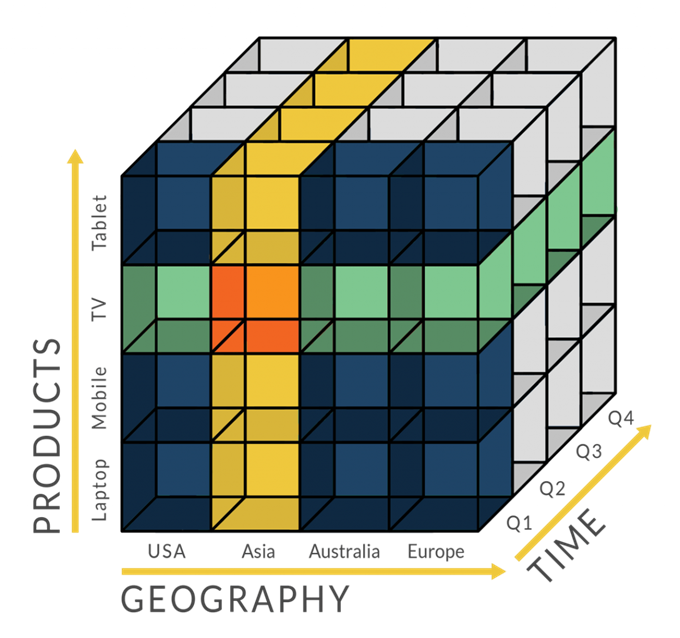# 数据分析之离散度指标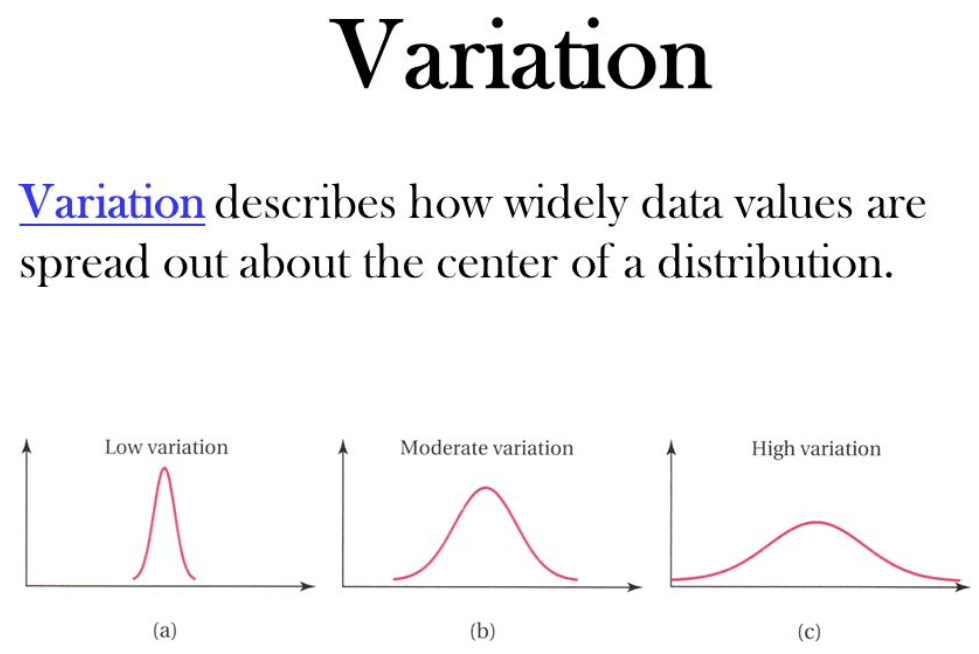$$R = x_{max} – x_{min}$$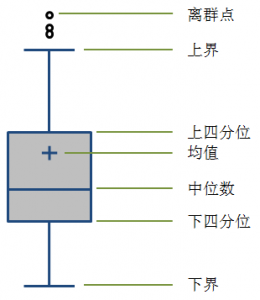$$IQR = Q3 – Q1$$

$$\sigma ^2 = \frac{\sum (x-\bar{x})^2}{n}$$

$$\sigma = \sqrt{\frac{\sum (x-\bar{x})^2}{n}}$$

$$MD = \frac{\sum \left | x – \bar{x} \right |}{n}$$

$$CV = \frac{\sigma }{\mu }$$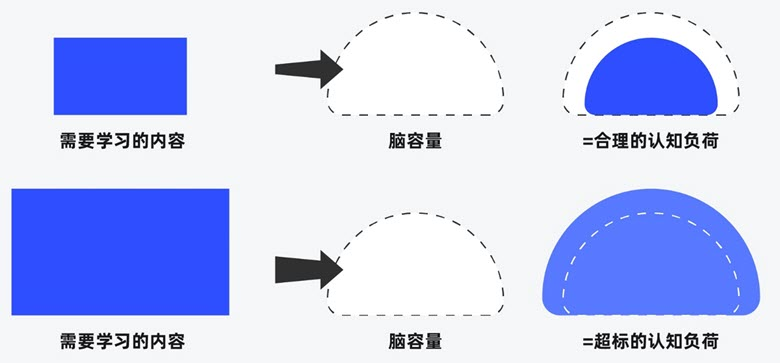##### 界面设计和游戏设计中的认知负荷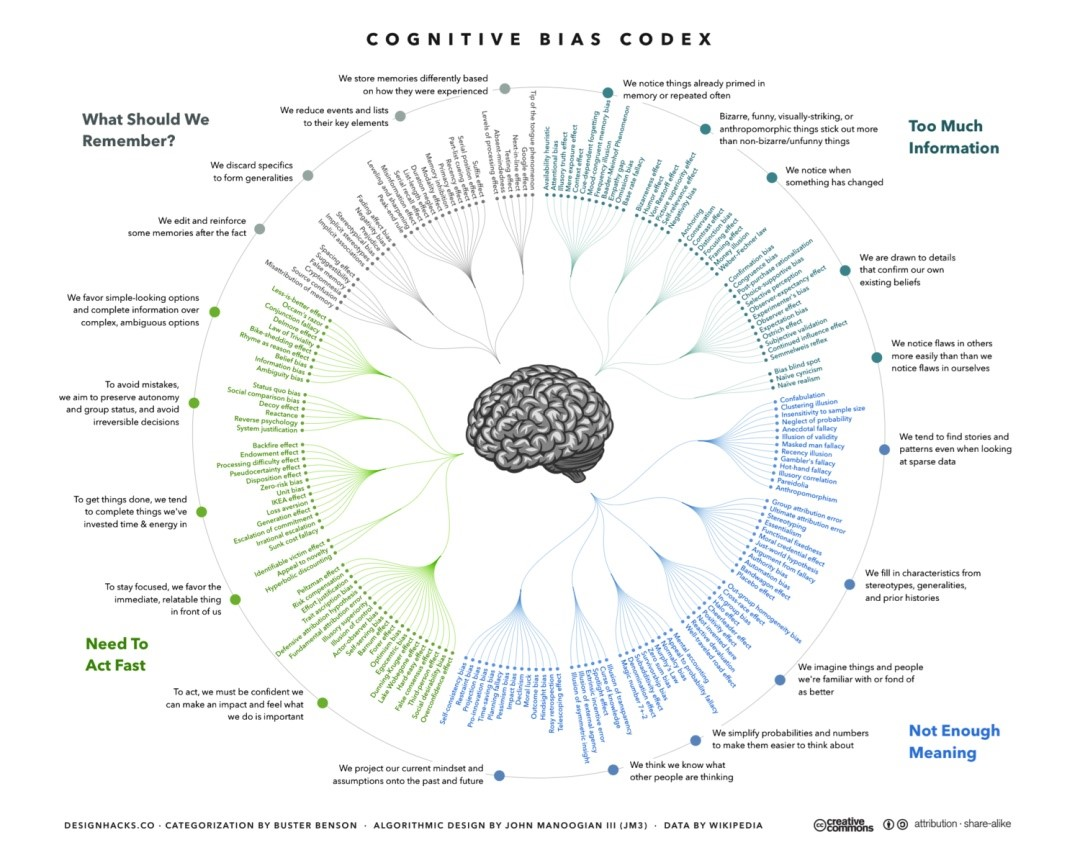##### 理解认知偏差